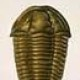# Mixed Quantitative Aptitude Questions Set 223

1. Train A leaves kolkata at 8am and is travelling at a speed of 70 km/hr, 4 hours later another train B leaves kolkata and is travelling in same direction as train A in how many time (in minutes) train B will be 25 km ahead train A, if speed of train B is 100 km/hr ?
475 minutes
610 minutes
340 minutes
620 minutes
368 minutes
Option B
Distance covered by train A in 4 hours = 70 * 4 = 280 km
total distance should be covered by train B from kolkata for ahead 25 km to train A
required time = 280 + 25/100-70 = 305/30 * 60 = 610 minutes

2. In a box there are 3 red balls, 7 white balls and 5 orange balls. If one bottle is drawn at random from the cartoon what is the probability that it is either red balls or white balls ?
2/7
8/9
3/4
5/7
2/3
Option E
probability = (3C1) + (7C1)/15C1 = (3 + 7)/15 = 2/3

3. Two persons A and B alone can do a piece of work in 10 days and 12 days respectively. A and B together worked for 4 days and remaining worked by C alone. If efficiency of C is 20% less than the efficiency of B then find in how many days total work will be completed ?
5 days
8 days
7 days
12 days
6 days
Option B
LCM of 10 and 12 = 60
efficiency of A = 60/10 = 6
efficiency of B = 60/12 = 5
work completed by A and B together in 4 days = (6 + 5)* 4 = 44 work
remaining work = 60 – 44 = 16 work
efficiency of C = 5 * 80/100 = 4
time required to complete the work = 16/4 = 4 work
total time to complete the work = 4 + 4 = 8 days

4. a and B invested rs.2500 and rs.3100 in a business respectively. After 6 months A left the business and C joined with a capital of rs.2200. At the end of 1.5 years the profit received by A and B together is how much percent more or less than that by C ?
125 3/8%
140 10/11%
48 3/5%
152 3/7%
215 3/8%
Option B
Profit sharing ratio of A , B and C = (2500 * 1.5) : (3100 * .5) : (2200 * 1) = 75 : 31 : 44
more % = (75 + 31) – 44/44 * 100 = 140 10/11%

5. Sudha is travelling from point A to B which is 560 km apart with a certain speed. While returning he covers half the distance with 30% less speed than the usual speed and cover the remaining distance with a speed of 140 km/hr. If time taken in both cases is equal, then find the speed of Sudha while going from point A to B ?
75 km/hr
80 km/hr
72 km/hr
84 km/hr
108 km/hr
Option B
Let speed of Sudha while travelling from point A to point B = 10x km/hr
time taken by Sudha while travelling from A to B = 560/10x
time taken by Sudha while returning = 280/7x + 280/140
560/10x = 280/7x + 2
56/x – 40/x = 2
x = 8
speed of Sudha = 10 * 8 = 80 km/hr

6. Directions : What will come in place of questions (?) marks in the following questions below ?
(15)^2 – (18)^2 + 312 = ? + 47
319
120
184
133
166
Option E
225 – 324 + 312 = ? + 47
– 99 + 312 = ? + 47
? = 166

7. ∛1331 + 38% of 1200 – ? = 98.20
421.50
213.40
368.80
254.20
281.20
Option C
11 + 456 – ? = 98.20
? = 368.80

8. 1/14 + 2/7 – 1/42 = ?
2/7
1/3
4/5
3/5
4/7
Option B
(3 + 12 – 1) /42 = ?
? = 1/3

9. 42.30 – (11)^2 + 520 – ? = 218.40
240.80
312.90
422.90
222.90
128.46
Option D
562.30 – 121 – ? = 218.40
? = 222.90

10. 3/4 of 420 + 320 – 4/5 of 215 = ? + 85
420
422
540
325
378
Option E
315 + 320 – 172 = ? + 85
? = 378

## 4 Thoughts to “Mixed Quantitative Aptitude Questions Set 223”

1.come funziona il sessodating

It’s in point of fact a nice and useful piece of
info. I’m glad that you simply shared this useful info with us.
Please keep us informed like this. Thank you for sharing.

2.¿qué opinan los usuarios sobre liruch

For latest information you have to pay a quick visit web and on web
I found this website as a best site for hottest updates.

3.KIU

935362 765281An extremely interesting examine, I may possibly possibly not concur entirely, but you do make some extremely valid points. 180620

4.40plusrelatie review

Wow, awesome blog layout! How long have you ever been blogging
for? you make blogging look easy. The entire look of your site is wonderful,
let alone the content material!